GCSE Maths Algebra Inequalities

Here we will learn about quadratic inequalities including how to solve quadratic inequalities, identify solution sets using inequality notation and represent solutions on a number line.

There are also quadratic inequalities worksheets based on Edexcel, AQA and OCR exam questions, along with further guidance on where to go next if you’re still stuck.

Quadratic inequalities are similar to quadratic equations and when plotted they display a parabola. We can solve quadratic inequalities to give a range of solutions.

For example,

The quadratic equation x^{2}+ 6x +5 = 0 has two solutions.

This is shown on the graph below where the parabola crosses the x axis.

We could solve this by factorising: (x + 1)(x + 5) = 0 .

The two values of x that equate this equation to zero are x = - 1 and x = - 5 .

For example,

The quadratic inequality x^{2}+ 6x +5  \leq 0 can be solved by factorising but instead of two solutions, there are a range of solutions.

x^{2}+ 6x +5 \leq 0 means that the we need to know the x values that when the graph is less than 0 .

This corresponds to where the curve is below the x axis.

We can see the from the graph that the x values need to be between -1 and -5 .

Therefore the solution to this inequality can be written using inequality notation,

-5\leq x \leq-1 .

For example,

The quadratic inequality x^{2} + 6x +5 > 0 is similar to the previous inequality and has a range of solutions.

x^{2}+ 6x +5 > 0 means that we need to know the x values when the graph is greater than 0

This corresponds to where the curve is above the x axis.

We can see the from the graph that the x values need to be greater than -1 and less than -5 .

Therefore the solution to this inequality can be written using inequality notation as two inequalities  x > -1 or x < -5 .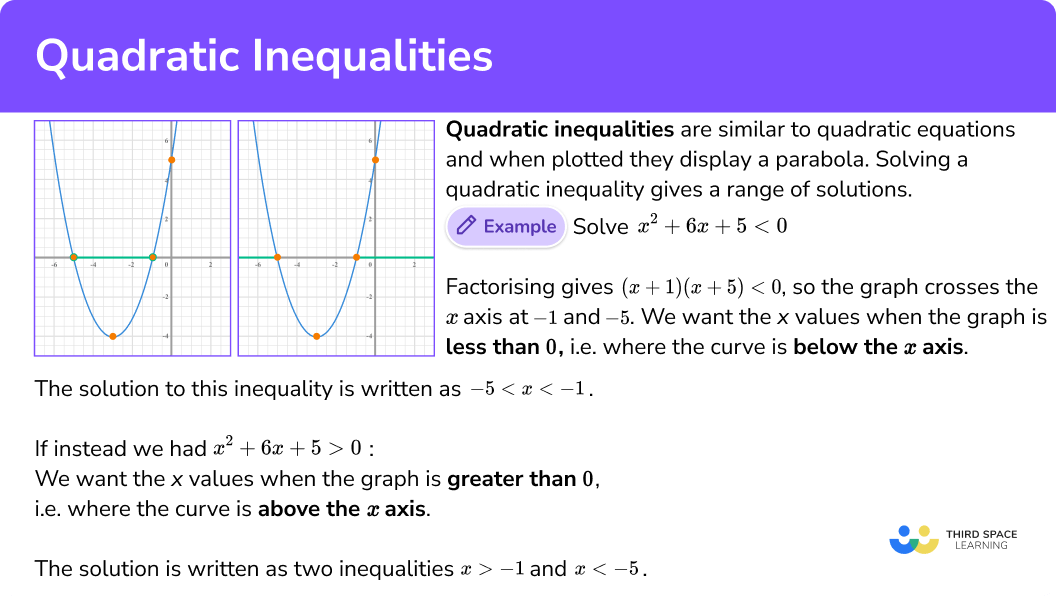## How to solve quadratic inequalities

In order to solve quadratic inequalities by factorising:

2. Find the values of x that make each bracket equal zero.
3. Write the solution using inequality notation.

1. Identify values of a, b and c to substitute into the quadratic formula.
3. Simplify to calculate the solutions of the inequality.
4. Write the solution using inequality notation.

### How to solve quadratic inequalities by factorising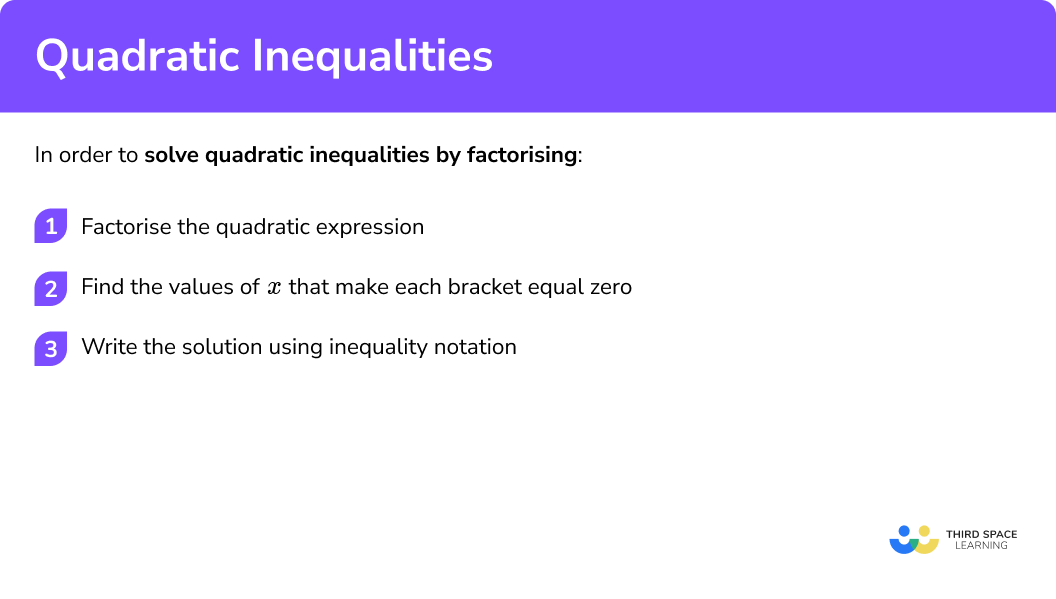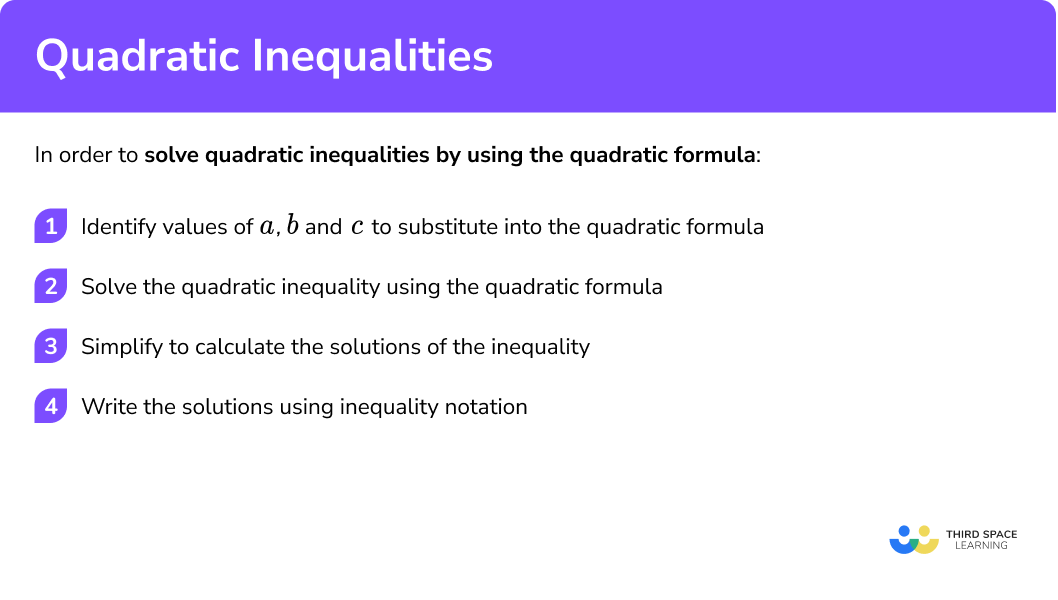## Related lessons on inequalities

Quadratic inequalities is part of our series of lessons to support revision on inequalities. You may find it helpful to start with the main inequalities lesson for a summary of what to expect, or use the step by step guides below for further detail on individual topics. Other lessons in this series include:

### Example 1: solving by factorising

Solve x ^{2}+7x+10 < 0 .

(x + 2)(x + 5) < 0

2Find the values of x that make each bracket equal zero.

(x + 2) = 0 when x = -2

(x + 5) = 0 when x = -5

3Write the solution using inequality notation.

We need the values of x that produce a graph that is less than 0 and so below the x axis.

The inequality is given by -5 < x < -2 .

4Represent the solution set on a number line.

-5 and -2 are not included in the solution set so these values are indicated with open circles.

### Example 2: solving by factorising

Solve x^{2}+3x+10\leq0 .

(x-2)(x+5)\leq0

(x - 2) = 0 when x = 2

(x + 5) = 0 when x = -5

We need the values of x that produce a graph that is less than or equal to 0 and so below the x axis.

The inequality is given by -5\leq x \leq2 .

-5 and 2 are included in the solution set so these values are indicated with closed circles.

### Example 3: solving by factorising

Solve x^{2}-6x+8\geq0 .

(x-2)(x-4)\geq0

(x -2) = 0 when x = 2

(x -4 ) = 0 when x = 4

We need the values of x that produce a graph that is greater than or equal to 0 and so above the x axis.

There will be two separate inequalities as there are two separate regions indicated on the graph.

x \geq4 and x \leq2 .

2 and 4 are included in the solution set so these values are indicated with closed circles.

### Example 4: solving by factorising

List the integers that satisfy 2x^{2} + 9x + 4\leq0

(2x+1)(x+4)\leq0

(2x + 1) = 0 when x = -\frac{1}{2}

(x +4 ) = 0 when x = -4

We need the values of x that produce a graph that is less than or equal to 0 and so below the x axis.

The inequality is given by -4\leq x \leq-\frac{1}{2} .

-4, -3, -2, -1

x is any value greater than or equal to  -4 and less than or equal to -\frac{1}{2} . The highest integer below -\frac{1}{2} is -1 .

### Example 5: solving by factorising

Solve x^{2}-9>0 .

(x -3)(x + 3) > 0

(x +3) = 0 when x = -3

(x -3 ) = 0 when x = 3

We need the values of x that produce a graph that is greater than 0 and so above the x axis.

There will be two separate inequalities as there are two separate regions indicated on the graph.

x>3 and x < -3 .

-3 and 3 are not included in the solution set so these values are indicated with closed circles.

### Example 6: solving by factorising and rearranging

List the integers that satisfy x^{2}<16 .

Rearrange the inequality so that it is less than 0 by subtracting 16 from both sides.

x^{2}-16< 0

(x - 4)(x + 4) = 0

(x +4) = 0 when x = -4

(x -4 ) = 0 when x = 4

We need the values of x that produce a graph that is less than 0 and so below the x axis.

The inequality is given by -4 < x < 4 .

-3, -2, -1, 0, 1, 2, 3

x is any value greater than -4 and less than 4 .

### Example 7: solving by using the quadratic formula

Solve 5x^{2}+6x-12\leq0 .

5x^{2}+6x-12\leq0

A quadratic expression is in the form ax^{2} + bx  + c .

In this inequality a = 5, b = 6 and c = -12 .

The quadratic formula is x = \frac{-b \pm \sqrt{b^{2}-4 a c}}{2 a} .

Substituting the values a = 5, b = 6 and c = -12 gives x = \frac{-6 \pm \sqrt{6^{2}-4 \times 5 \times-12}}{2 \times 5} .

We need to use the quadratic formula to solve rather than factorising as the discriminant is not a square number.

$x = \frac{-6 \pm \sqrt{6^{2}-4 \times 5 \times-12}}{2 \times 5} \\ x = \frac{-6 \pm \sqrt{36–240}}{10} \\ x = \frac{-6 \pm \sqrt{276}}{10}$

$x= \frac{-6+2\sqrt{69}}{10} \quad and \quad x=\frac{-6-2\sqrt{69}}{10}\\ x= \frac{-3+\sqrt{69}}{5} \quad and \quad x=\frac{-3-\sqrt{69}}{5}$

In this case the answer is given in surd form but you may be asked to leave the answers rounded to a degree of accuracy.

We need the values of x that produce a graph that is less than or equal 0 and so below the x axis.

The inequality is given by \frac{-3- \sqrt{69}}{5} \leq x \leq \frac{-3+ \sqrt{69}}{5} .

### Example 8: solving by using the quadratic formula

Solve 2x^{2}-3x- 4 < 0 .

2x^{2}-3x- 4 < 0

A quadratic expression is in the form ax^{2}+bx+c .

In this inequality a = 2, b = -3 and c = -4 .

The quadratic formula is x = \frac{-b \pm \sqrt{b^{2}-4 a c}}{2 a} .

Substituting the values   a = 2, b =-36 and c = -4 gives

x=\frac{-(-3) \pm \sqrt{(-3)^{2}-4 \times 2 \times-4}}{2 \times 2} .

$x=\frac{-(-3) \pm \sqrt{(-3)^{2}-4 \times 2 \times-4}}{2 \times 2} \\ x=\frac{3 \pm \sqrt{9–32}}{4} \\ x=\frac{3 \pm \sqrt{41}}{4}$

$x=\frac{3+6.4}{4} \quad and \quad x=\frac{3-6.4}{4}\\ x = 2.35 \quad and \quad x = -0.85$

In this case the answer is being given to two decimal places but you could leave the solution in surd form.

We need the values of x that produce a graph that is less than 0 and so below the x axis.

The inequality is given by -0.85 < x< 2.35 .

Rounded to two decimal places.

### Example 9: one solution

Solve x^{2}+6x+9\leq0 .

(x + 3)(x + 3) \leq 0 or (x+3)^{2}

As both brackets are equal, there is only one solution.

(x +3) = 0 when x = -3

We need the values of x that produce a graph that is less than or equal to 0 and so below the x axis. This only happens when, x = -3 .

### Common misconceptions

• Square root of a number has two solutions

A common error when calculating the square root of a number is only writing the positive solution. A negative number squared will also give a positive solution.

For example,

When solving x^2=16, x=\pm \sqrt{16} =\pm 4 .

• Not maintaining the original inequality sign

A common error is to accidentally change the direction of the inequality sign or not writing an inequality sign in the solution.

• Writing a double inequality when the solution set is split

When solving  x^{2} + 10x +16  < 0 there is one solution set which is below the x axis. Therefore the solution is -8 < x < -2 .

When solving  x^{2} + 10x +16  > 0 there is one solution set which is above the x axis. Therefore the solution is x<-8 and x > -2 .

1. Solve x^{2}+13x+30<0 .

-10 <x< -310 <x<3-10 and -3-10 >x> -3x^{2} + 13x +30 < 0

Factorise the quadratic, (x + 3)(x +10) < 0 .

Find the values of x that make the bracket equal zero, x = -10 and x = -3 .

Write the solution using interval notation, -10 < x < -3 .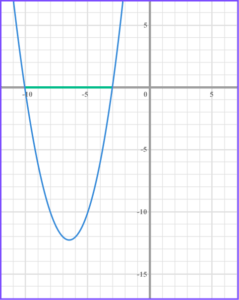2. Solve x^{2}+3x-10\leq0 .

5\leq x \leq-2-5\leq x \leq 2-5 and -2-5\leq x \leq -2x^{2}+3x-10 \leq 0

Factorise the quadratic, (x + 5)(x -2) \leq 0 .

Find the values of x that make the bracket equal zero, x = -5 and x = 2 .

Write the solution using interval notation, -5\leq x \leq2 .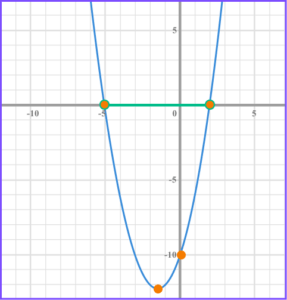3. Solve x^{2}-7x+6\leq0 .

1 \leq x < 62 \geq x \geq 31 \geq x \leq 6x \leq 1 and x\geq 6x^{2}-7x+6\geq 0

Factorise the quadratic, (x-6)(x-1)\geq 0 .

Find the values of x that make the bracket equal zero, x = 6 and x =1 .

Write the solution using interval notation, x \leq 1 and x\geq6 .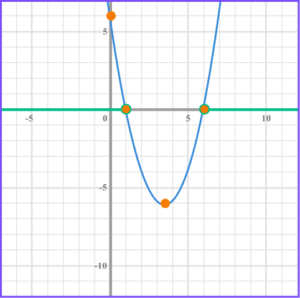4. Solve 2x^{2}-11x+ 5 \leq 0 .

-5\leq x \leq -\frac{1}{2}1\leq x \leq 5\frac{1}{2}\leq x \leq 5-\frac{1}{2}\leq x \leq 52x^{2}-11x+ 5 \leq 0

Factorise the quadratic, (2x-1)(x-5) \leq 0 .

Find the values of x that make the bracket equal zero, x =\frac{1}{2} and x =5 .

Write the solution using interval notation, \frac{1}{2} \leq x\leq 5 .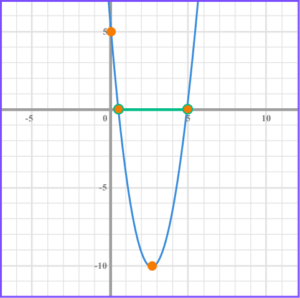5. Solve x^{2}-25>0 .

-5\leq x \leq 5x = -5 and x =5-5 > x > 5x > 5x^{2} – 25 > 0

Factorise the quadratic, (x + 5)(x -5) > 0 .

Find the values of x that make the bracket equal zero, x = -5 and x =5 .

Write the solution using interval notation, x < -5 and x > 5 .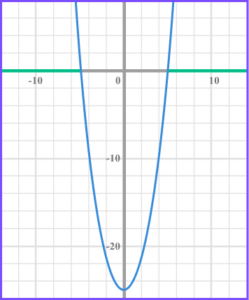6. Solve   x^{2}<1 .

-1 < x < 1-1 > x > 1x < -1-1\leq x \leq 1x^{2} < 1

Rearrange to make the inequality less than zero, x^{2} – 1<0 .

Factorise the quadratic, (x + 1)(x -5) < 0 .

Find the values of x that make the bracket equal zero, x = -1 and x =1 .

Write the solution using interval notation, -1 < x < 1 .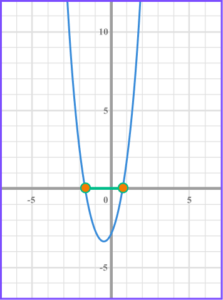7. Solve 3x^{2}+4x-2\leq 0 .

-0.39\leq x \leq 1.72-1.72\geq x \leq 0.39-1.72\leq x \leq 0.39-3\leq x \leq 43x^{2} + 4x – 2 \leq 0 .

Identify a, b and c from the form ax^{2} +bx + c \leq 0 , a = 3, b = 4, c = -2 .

Substitute the values in to the quadratic formula, x = \frac{-4 \pm \sqrt{4^{2}-4 \times 3 \times-2}}{2 \times 3} .

Simplify the inequality, x = \frac{-4 \pm \sqrt{16–24}}{6} .

Simplify further, x=\frac{-4 \pm \sqrt{40}}{6} .

You could simplify to surd form, x = \frac{-4 \pm 2 \sqrt{10}}{6} .

Simplify the fraction. You could leave in surd form, x = \frac{-2 \pm \sqrt{10}}{3} .

Correct to 2 decimal places, x \leq 0.39 and x \geq -1.72 .

Written in surd form written in interval notation, \frac{-2+\sqrt{10}}{3} \leq x \leq \frac{-2+\sqrt{10}}{3} .

Written as an inequality with values rounded to 2 decimal places, -1.72 \leq x \leq 0.39 .8. Solve x^{2}-10x+25 \leq 0 .

x = 5-5\leq x \leq -\frac{1}{2}-5 >x > 5x\leq5x^{2} -10x +25 \leq 0 .

Factorise the quadratic, (x – 5)(x -5) \leq 0 .

Find the values of x that make the bracket equal zero, x =5 .

The quadratic only has one root at 5, so is never less than zero but equal to zero at 5, x = 5 .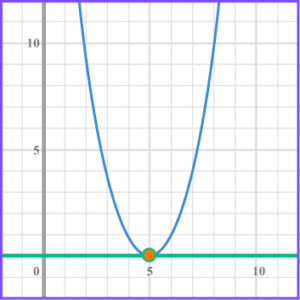1. Solve the inequality x^{2}-8x+15\leq 0 .

(4 marks)

(x-3)(x-5) \leq 0

Factorise the inequality. 1 mark if there are one or two errors.

(2)

Roots found x = 3 and x = 5

(1)

3\leq x \leq 5

(1)

2. (a) Find the interval for which x^{2}+3x – 10 < 0 .

(b) Represent your solution to part (a) on the number line below.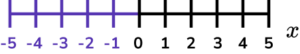(6 marks)

(a)

(x -2)(x + 5) < 0

1 mark if there are one or two errors.

(2)

Roots found x =2 and x = -5

(1)

-5 < x < 2

(1)

(b)

-5 and 2 indicated.

(1)

Correct solution with open circles.(1)

3. Here is a rectangle.All measurements are in centimetres.

x is an integer.

The total area of the rectangle is less than 21cm^{2} .

Show that 4 <x < 7 .

x^{2} – 4x <21 .

(1)

x^{2} – 4x – 21< 0 .

(1)

(x – 7)(x + 3) < 0 .

(1)

7 and -3

(1)

4<x < 7 as you cannot have a negative value for distance.

(1)

## Learning checklist

You have now learned how to: